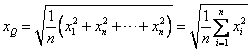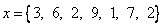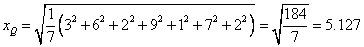Quadratic mean is useful indicator of many performance measurements. It is a square root of average of sum of square.

Other name: root mean sum of square or root mean square (RMS)

Formula:Notes:

Example:Try the interactive program below to compute Quadratic mean. Feel free to change with your own input values.

Input list of numbers separated by comma, then press the button "Get Quadratic Mean". The program will compute directly when you change the input data.

Preferable reference for this tutorial is

Teknomo, Kardi (2015) Mean and Average. https:\\people.revoledu.com\kardi\ tutorial\BasicMath\Average\